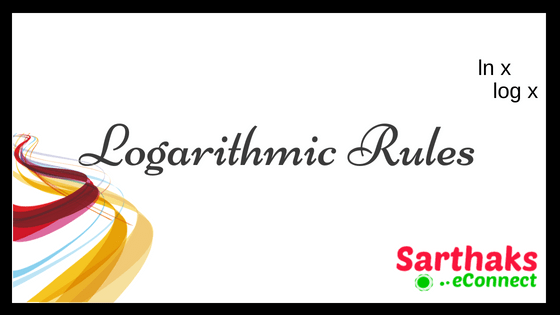# Log rules:

In dealing with various types of problems in almost every section of mathematics we see there is use of log rules. As we see in algebra, exponents, calculus or in simple number system we have to use logarithm property for calculation.

## Basic Log rules:

As we know that logarithm is the inverse function of exponentiation. This is the basic of log rules and is explained as:
logax is the inverse function of the exponential function ax.

Thus if logac = k thus can be resolved to ak = c
when we talk of natural logarithm, It is essential to note that
logex = ln x
There should not be any confusion regarding natural logarithm and common logarithm. Only the difference is the difference of base. Common logarithm has a base of 10 whereas natural logarithm has a base of e.

## Special log rules:

### The product rule:

We use the product log rules for exponentiation to derive a corresponding product rule for logarithms. Using the base b=e, the product rule for exponentials is

eaeb = ea+b
eln(xy) = xy ,Where xy can be written as eln xeln y

Also, we can write eln x + ln YThus, we can say ln(xy) = ln(x) + ln(y)

### The quotient rule:

While reading about log rules we must understand quotient rule as well as its applications.

As we know for exponential base:

$$\frac{{e}^a}{{e}^b}$$ = ea-b

Thus we can define quotient logarithmic rule as

ln( $$\frac{x}{y}$$ ) = ln(x) – ln(y)

### Log of a power:

First to understand about power pattern of the logarithmic function we will deal with exponential function. The logarithmic rules for log of power is as stated:

(ea)b = eab
After deriving the same on the function of x and y we aspired that

ln(xy) = y ln(x)

we call this rule as the rule of power.

### Log of e:

As we are aware that if any real number has the power 1 than it consists the same value itself.
e1 = e
By taking logarithm on both side we get
ln(e) = 1

### Log of 1:

Any real number containing power zero has a value of 1. So it describes log rules as
e0 = 1
By taking logarithm on both side we get,
ln(1) = 0

### Log of reciprocal rule:

Any real number with negative power becomes reciprocal of the number itself. Thus logarithm rules can be described as:
x-1 = $$\frac{1}{x}$$
As we know that from power rule
ln(xy) = y ln(x)
simply we put y = -1 in the above equation.

we get,
ln( $$\frac{1}{x}$$ ) = – ln(x)

### Log rules for base switch:

Base to exponent in reciprocal system of logarithm takes place by this rule:
logb(c) = $$\frac{1}{log_{c}(b)}$$

### Log rules for base change:

This rule is very important as we require it every time.
logax = $$\frac{log_{b}(x)}{log_{b}(a)}$$ = $$\frac{ log\:x}{log\:a}$$

## Some important points related to log rules:

1. Logarithm function is denoted by (x) = logb(x) where b is base of logarithm.
2. Derivative of logb(x) is $$\frac{1}{xlnb}$$.
3. The limit of the log of x with base a, when x approaches infinity, is equal to infinity.
4. Integral or anti-derivative of logb(x) is x.(logb(x) – $$\frac{1}{lnb}$$ ) + c